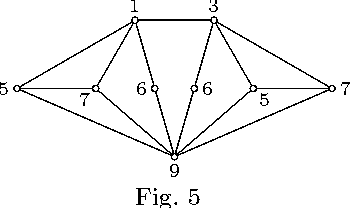You are currently offline. Some features of the site may not work correctly.

# Hamiltonian coloring

Hamiltonian coloring is a type of graph coloring. Hamiltonian coloring uses a concept called detour distance between two vertices of the graph. It… Expand
Wikipedia

## Papers overview

Semantic Scholar uses AI to extract papers important to this topic.
2017
2017
• D. Bantva
• J. Graph Algorithms Appl.
• 2017
• Corpus ID: 16784185
Let $G$ be a simple connected graph of order $n$. A hamiltonian coloring $c$ of a graph $G$ is an assignment of colors (non… Expand
2016
2016
A hamiltonian coloringc of a graph G of order n is a mapping c : $$VG \rightarrow \{0,1,2,...\}$$ such that Du,i¾źv + |cu - cv… Expand
2016
2016
A hamiltonian coloring c of a graph G of order p is an assignment of colors to the vertices of G such that D(u, v) + \(|c(u) - c… Expand
2012
2012
• Discuss. Math. Graph Theory
• 2012
• Corpus ID: 26560540
For a strong oriented graph D of order n and diameter d and an integer k with 1 ≤ k ≤ d, the kth power D k of D is that digraph… Expand
•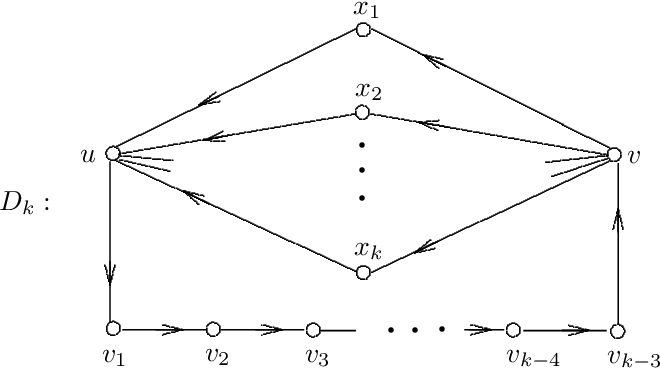•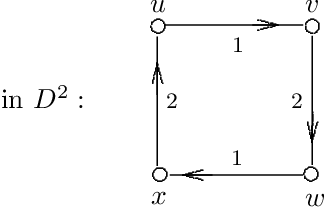•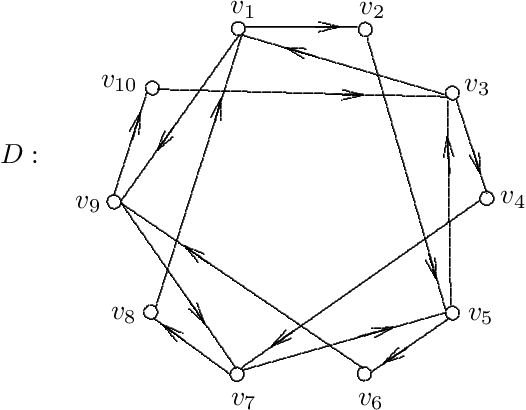•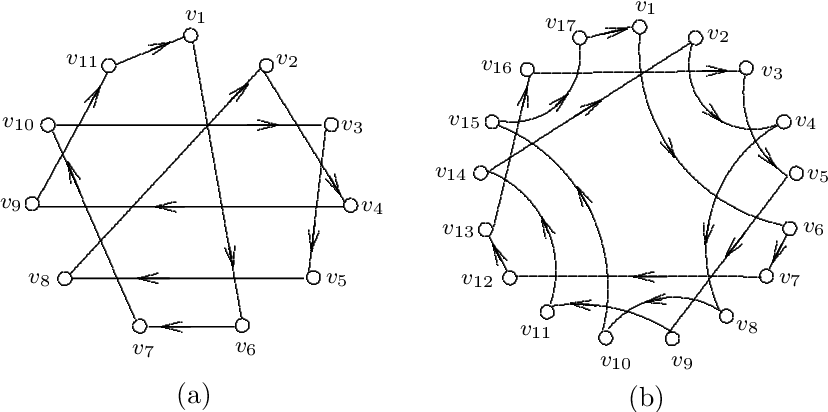•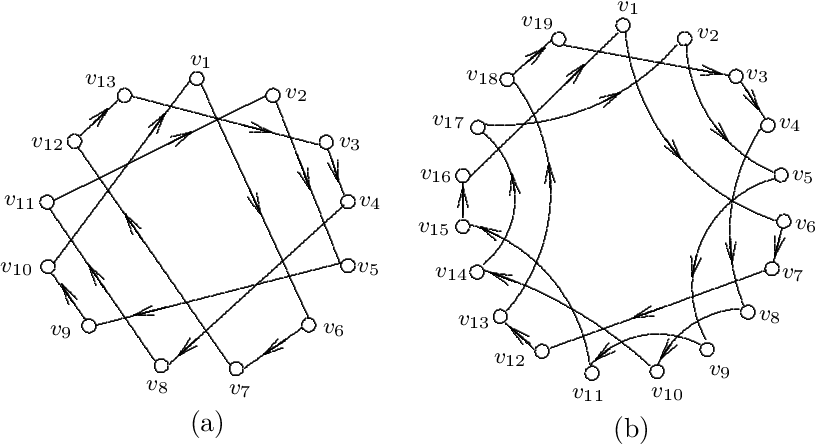2008
2008
• Discret. Appl. Math.
• 2008
• Corpus ID: 45500058
For a connected graph G and any two vertices u and v in G, let D(u,v) denote the length of a longest u-v path in G. A hamiltonian… Expand
2005
2005
• Discret. Math.
• 2005
• Corpus ID: 207130615
A hamiltonian coloring of a connected graph G of order n is an assignment c of colors (positive integers) to the vertices of G… Expand
•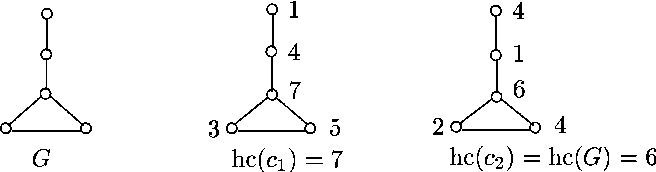2003
2003
By a hamiltonian coloring of a connected graph G of order n > 1 we mean a mapping c of V (G) into the set of all positive… Expand
•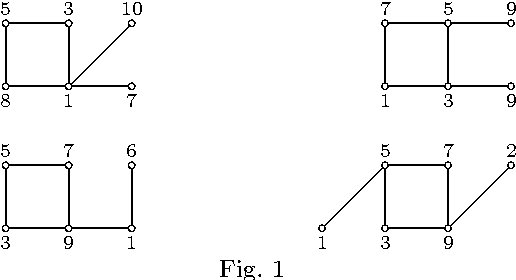•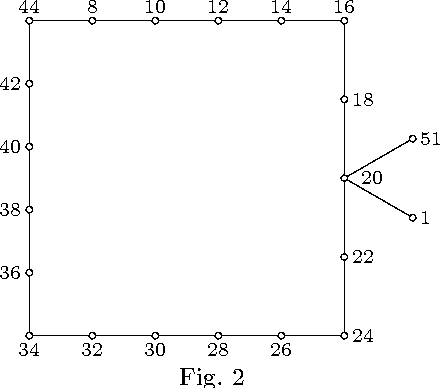•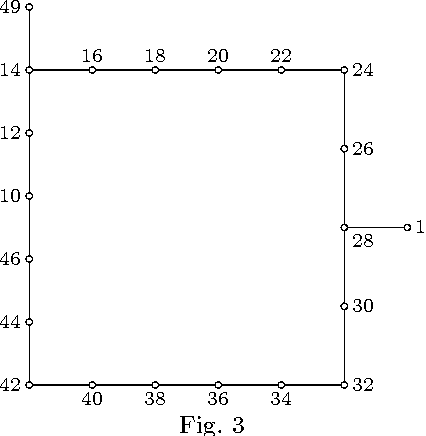•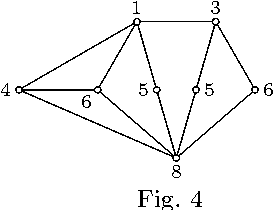•